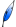# Softwares

### Plugin FIGARO (Imagej)

This plugin FIGARO for the open-source ImageJ software, helps tracking resolution of microscope systems by extracting dimensions and orientation of standardized microbeads images, acquired from test samples. In the development of optical microscopes for biomedical imaging, the evaluation of resolution is a fundamental parameter achieved by Point Spread Function (PSF) measurements. Sometimes, PSF measurement procedure is not easy or impossible in case of microspheres images presenting a high noise level. The current method proposed into the plugin FIGARO relies on a variational approach for PSF modeling through multivariate Gaussian fitting, adapted to images acquired in a high noise context, described here.

Download : here

### The Proximity Operator Repository

Proximity operators have become increasingly important tools as basic building blocks of proximal splitting algorithms, a class of algorithms that decompose complex composite convex optimization methods into simple steps involving one of the functions present in the model. The website available here provides formulas for efficiently computing the proximity operator of various functions, along with the associated codes.

### Matlab toolbox for parallel MRI data reconstruction with the Majorize-Minimize Memory Gradient Algorithm

Download : ToolboxPMRI_v1.0.tar.gz ; ToolboxPMRI_v1.0.zip

This Matlab toolbox allows to solve the complex-valued image reconstruction problem arising in Parallel Magnetic Resonance Imaging (pMRI). The considered criterion is composed with a least square function as data fidelity term, and a regularization term favorizing the sparsity of the details coefficients of an orthogonal wavelet decomposition of the image. The reconstruction process uses the Majorize-Minimize Memory Gradient Algorithm described here.

### Matlab toolbox for linear unmixing with the Interior Point Least Squares algorithm

Download: IPLS_v1.0.tar.gz ; IPLS_v1.0.zip

This Matlab toolbox allows to solve the quadratic programming problem arising in the linear unmixing of hyperspectral data. A least squares criterion is minimized, under one of the following constraints: positivity ; positivity and sum equal to one ; positivity and sum less than one. The unmixing process uses the Interior Point Least Squares algorithm described here.

### Matlab toolbox for image restoration with the Variable Metric Forward-Backward algorithm

Download: RestoVMFB_Lab_v1.0.tar.gz ; RestoVMFB_Lab_v1.0.zip
For more informations:This Matlab toolbox allows to restore an image degraded by a linear operator and Gaussian Dependant noise with variance depending linearly on the image. The considered criterion is composed with the neg-log-likelihood of the noise distribution as data fidelity term, the indicator function allowing to constraint the dynamic range and the isotropic total variation favorizing piecewise constant images. The restoration process uses the Majorize-Minimize Variable Metric Forward-Backward Algorithm described here.

### Matlab toolbox for image restoration with the Majorize-Minimize Memory Gradient Algorithm

Download: RestoMMMG_Lab_v1.0.tar.gz ; RestoMMMG_Lab_v1.0.zip
For more informations:This Matlab toolbox allows to restore an image degraded by a linear operator and Gaussian noise. The considered criterion is composed with a least square function as data fidelity term, a quadratic distance function allowing to constraint the dynamic range of the restored image into [xmin,xmax], a quadratic elastic net term allowing to ensure the existence of the solution and a regularization term favorizing piecewise constant images. The restoration process uses the Majorize-Minimize Memory Gradient Algorithm described here.

### Matlab toolbox for linesearch on logarithmic barrier function

Download: LSMM_v1.0.tar.gz ; LSMM_v1.0.zip
For more informations:This Matlab toolbox allows to determine a suitable stepsize in iterative descent algorithms applied to the minimization of a criterion containing a logarithmic barrier function associated to linear constraints. The Majorize-Minimize (MM) algorithm described here is adopted. It relies on the derivation of a log-quadratic majorant function well suited to approximate the criterion containing barrier terms. The convergence of classical descent algorithms when this linesearch strategy is employed, is ensured. A demo file illustrates the efficiency of the MM linesearch on the Newton minimization of the barrier criterion associated to a random quadratic programming (QP) test problem.